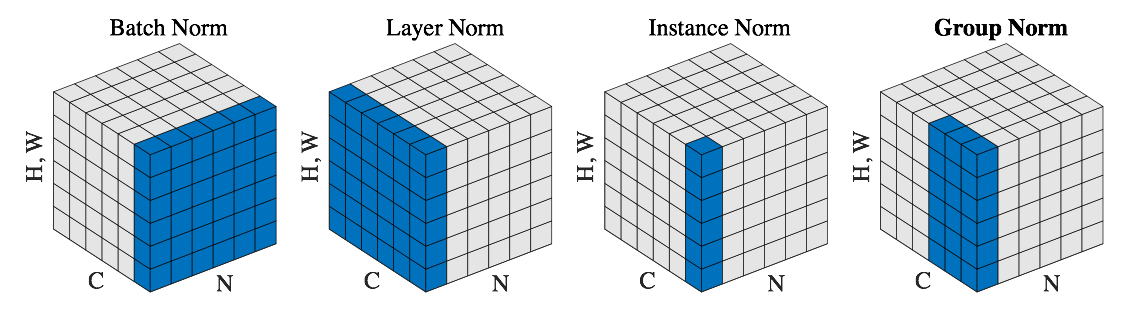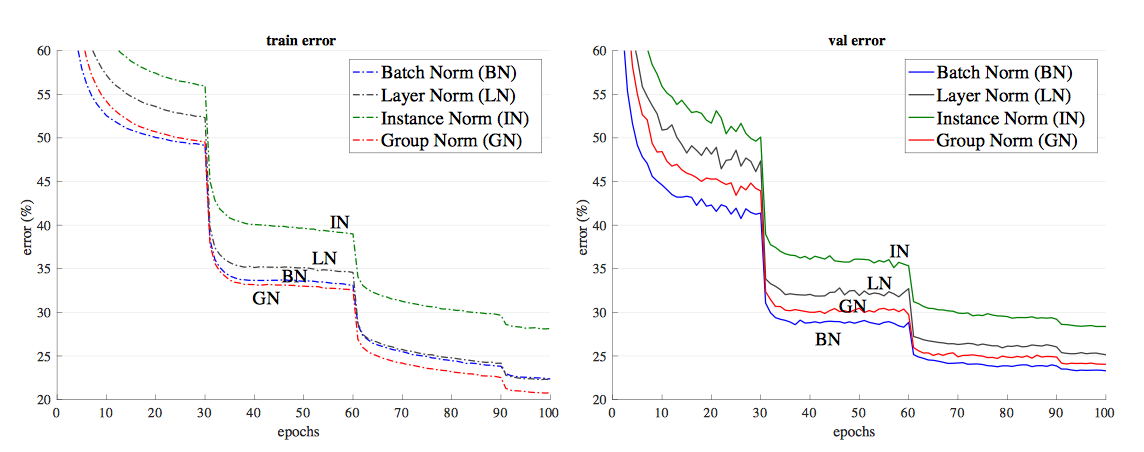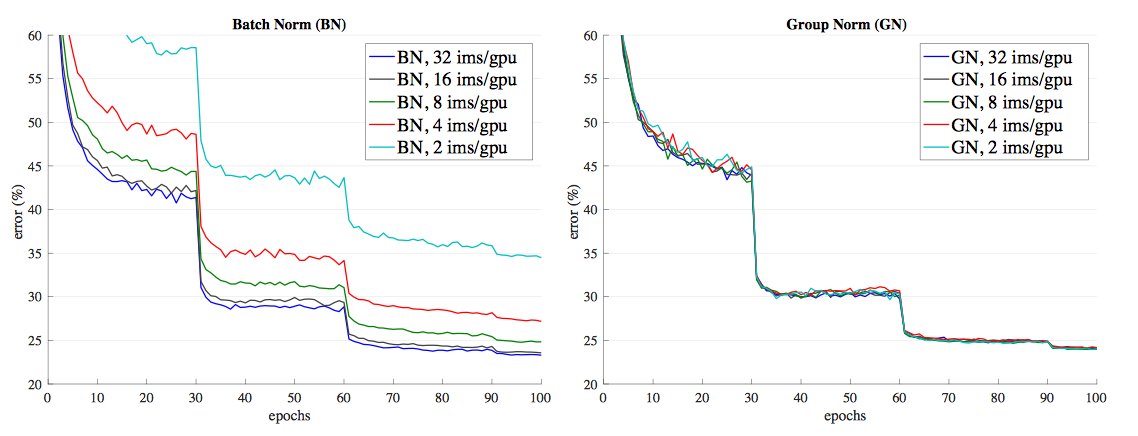Prince Home Stay Follish, Stay Hungry

# Group NormalizationGroup Normalization是今年何凯明大神的新作，抽时间拜读了一下，收获良多，也给了我在normalization和group conv上的一些新的理解，由于篇幅原因，在这篇博客中没有添加分组卷积的内容，如果有需要以后会继续补充。

## BN存在的问题

BN大法虽然好，但是仍存在着一些问题：

1. BN只在训练过程中起作用
2. BN在Batch的时候，梯度计算不稳定

The normalization of activations that depends on the mini-batch allows efﬁcient training, but is neither necessary nor desirable during inference; we want the output to depend only on the input, deterministically. For this, once the network has been trained, we use the normalization

## 与其他规范化方法的关系

### 插播• Batch Normalization(BN)

• Layer Norm(LN)

• Instance Norm(IN)

• Group Norm(GN)

GN这种思想借鉴了目前比较流行的Group Convolution的思想，认为相邻的通道表示的特征可以有一致性。文章后面通过实验证明了，GN在大Batch训练师在性能上能够接近BN的效果，而在小batch的时候能够和大Batch拥有几乎一样的性能。

## 实现

### Tensorflow实现

def GroupNorm(x, gamma, beta, G, eps=1e−5):
# x: input features with shape [N,C,H,W]
# gamma, beta: scale and offset, with shape [1,C,1,1]
# G: number of groups for GN
N, C, H, W = x.shape
x = tf.reshape(x, [N, G, C // G, H, W])
mean, var = tf.nn.moments(x, [2, 3, 4], keep dims=True)
x = (x − mean) / tf.sqrt(var + eps)
x = tf.reshape(x, [N, C, H, W])
return x ∗ gamma + beta


### PyTorch官方实现

 class GroupNorm(Module):
def __init__(self, num_groups, num_channels, eps=1e-5, affine=True):
super(GroupNorm, self).__init__()
self.num_groups = num_groups
self.num_channels = num_channels
self.eps = eps
self.affine = affine
if self.affine:
self.weight = Parameter(torch.Tensor(num_channels))
self.bias = Parameter(torch.Tensor(num_channels))
else:
self.register_parameter('weight', None)
self.register_parameter('bias', None)
self.reset_parameters()

def reset_parameters(self):
if self.affine:
self.weight.data.fill_(1)
self.bias.data.zero_()

def forward(self, input):
return F.group_norm(
input, self.num_groups, self.weight, self.bias, self.eps)


PyTorch中F.group_norm暂时还没找到在哪实现的，不过应该和tf的实现差不多，其实就是Reshape+norm的一个操作，然后就是一个BN经典的重分布操作（$\gamma x + \beta$）使分布可学习。

## 实验